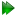The universal solution U

The complex model-free problem, in which the motions of each spin are both mathematically and statistically dependent on the diffusion tensor and vice versa, was formulated using set theory in d'Auvergne and Gooley (2007). This paper is important for understanding the entire concept of the new protocol in relax and for truly grasping the complexity of the model-free problem. The solutionto the model-free problem was derived as an element of the universal set, the union of the diverse model-free parameter spaces. Each setwas constructed from the union of the model-free modelsfor all spins and the diffusion parameter set. A single parameter loss on a single spin shifts optimisation to a different space. Ever since the seminal work of Kay et al. (1989) the model-free problem has been tackled by first finding an initial estimate of the diffusion tensor and then determining the model-free dynamics of the system (see Sections 7.5 on pageand 7.6 on page). This diffusion seeded paradigm is now highly evolved and much theory has emerged to improve this path to the solution. The technique can, at times, suffer from a number of issues including the two minima problem of the spheroid diffusion tensor parameter space, the appearance of artificial chemical exchange (Tjandra et al., 1996), the appearance of artificial nanosecond motions (Schurr et al., 1994), and the hiding of internal nanosecond motions caused by the violation of the rigidity assumption (Orekhov et al., 1999b,1995,1999a).

The relax user manual (PDF), created 2020-08-26.